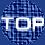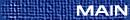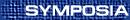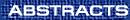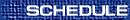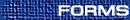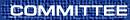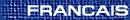CMS Jeffery-Williams Lecture / Conférence Jeffery-Williams de la SMC
(David Boyd, Organizer)

DAVID BOYD, University of British Columbia, Vancouver, British Columbia
Mahler's measure, hyperbolic geometry and the dilogarithm

If P(x,y) is a polynomial with integer coefficients, then the Mahler measure m(P) is defined to be the average of log|P| over the torus. That is

 m(P) = óõ 1 0 óõ 1 0 log|P(e2pi s,e2pi t)| ds  dt.
The starting point of our lecture will be the remarkable formula of Smyth, proved in the late 1970's:

 m(1 + x + y) = 3 Ö34p L(c-3,2),
where L(c-3,s) is the Dirichlet L-series for the odd character of conductor 3.

Chinburg asked the natural question whether there are other similar formulas for other Dirichlet characters. We will show how this question is connected with hyperbolic geometry and to K-theory via the theory of the Bloch group, and use this to produce many similar formulas (some conjectural and some proved) involving Dirichlet L-functions and L-functions of elliptic curves.